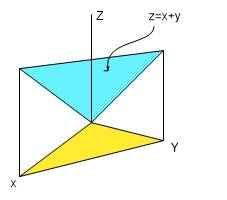# How to determine the volume of a region bounded by planes?

## Homework Statement

Let G be the region bounded by the planes x=0,y=0,z=0,x+y=1and z=x+y.

## Homework Equations

(a) Find the volume of G by integration.
(b) If the region is a solid of uniform density, use triple integration to find its center of mass.

## The Attempt at a Solution

[/B]
My understanding is that I need to setup a triple integral:

∫∫∫dxdydz

I’m just a little unsure about how to determine the terminals

mfb
Mentor
Did you draw a sketch?

You can always find boundaries for e.g. z as function of x,y and boundaries for y as function x, but sometimes there are easier methods.

LCKurtz
Homework Helper
Gold Member
Have you drawn a picture? That's the first step. Maybe this will help:#### Attachments

Did you draw a sketch?

You can always find boundaries for e.g. z as function of x,y and boundaries for y as function x, but sometimes there are easier methods.
So would the boundaries be:

0≤z≤x+y
0≤y≤1-x
0≤x≤1

mfb
Mentor
That will work.